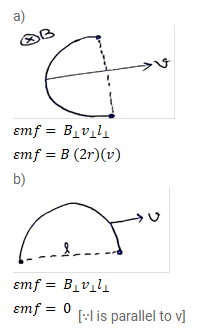# A copper wire bent in the shape of a semicircle of radius r

Question:

A copper wire bent in the shape of a semicircle of radius $r$ translates in its plane with a constant velocity v. A uniform magnetic field $B$ exists in the direction perpendicular to the plane of wire. Find the emf induced between the ends of the wire if (a) the velocity is perpendicular to the dimeter joining free ends, (b) the velocity is parallel to this diameter.

Solution: